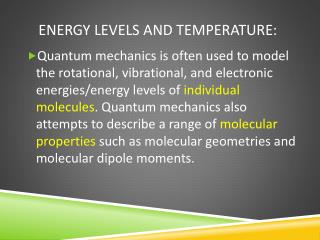DownloadDownload PresentationEnergy Levels and Temperature:

# Energy Levels and Temperature:

Télécharger la présentation## Energy Levels and Temperature:

- - - - - - - - - - - - - - - - - - - - - - - - - - - E N D - - - - - - - - - - - - - - - - - - - - - - - - - - -
##### Presentation Transcript

1. Energy Levels and Temperature: • Quantum mechanics is often used to model the rotational, vibrational, and electronic energies/energy levels of individual molecules. Quantum mechanics also attempts to describe a range of molecular properties such as molecular geometries and molecular dipole moments.

2. Energy levels and temperature: • Classical thermodynamics deals with the macroscopic properties of matter (S, H, H, Cv and so on). In higher level chemistry courses statistical mechanics is employed to link quantum mechanics and thermodynamics.

3. Statistical Thermodynamics: • Key idea: If one can describe the energy level patterns of individual atoms or molecules then the properties of a macroscopic system (many molecules) should be “readily” calculated. As temperature increases molecules are more likely to “occupy” higher energy levels.

4. Spectra and energy level population: • The different populations of energy levels manifest themselves in many ways including the intensities of spectral lines – these line intensities are temperature dependant. The Boltzmann expression can help us here and later in the description of lasers.

5. Boltzmann Expression:

6. Boltzmann Distribution: • Here n1 and n2 specify the relative numbers of molecules in energy levels 1 and 2, g1 and g2 are the degeneracies of the two levels, ϵ1 and ϵ2 are the energies of the two levels ( in J per molecule), kB is the Boltzmann constant and T is the Kelvin temperature.

7. Boltzmann Expression:

8. MOLECULAR partition Function:

9. Molecular Partition Function: • Partition functions “contain” all of the thermodynamic information about a system of independent molecules at equilibrium. We will leave the q → U, H, S, CV calculations for another course and focus on understanding how populations of levels change as T increases/decreases.

10. Molecular Partition Function:

11. Molecular Partition functionS:

12. Molecular Partition Function:

13. Molecular Partition Functions:

14. Molecular Partition Functions:

15. Boltzmann and partition functions: • Class example: We will examine the simple case of 2 energy levels: ϵ0 = 0 and ϵ1 = ϵ. We can evaluate all quantities as a function of kBT. If, for example, kBT = 2ϵ then kBT/ϵ = 2. We will write equations for the partition function and energy levels populations and plot graphs with the data on the next slide.

16. Two Energy Level system Populations: